## Torsion (Differential Geometry)

The rate of change of the Osculating Plane of a Space Curve. The torsion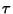is Positive for a right-handed curve, and Negative for a left-handed curve. A curve with Curvature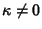is planar Iff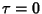.

The torsion can be defined by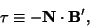where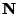is the unit Normal Vector and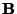is the unit Binormal Vector. Written explicitly in terms of a parameterized Vector Function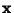,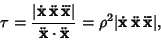where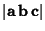denotes a Scalar Triple Product and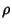is the Radius of Curvature. The quantity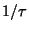is called the Radius of Torsion and is denoted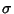or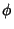.

Gray, A. Drawing Space Curves with Assigned Curvature.'' §7.8 in Modern Differential Geometry of Curves and Surfaces. Boca Raton, FL: CRC Press, pp. 145-147, 1993.
Kreyszig, E. Torsion.'' §14 in Differential Geometry. New York: Dover, pp. 37-40, 1991.